Courses

# Fill Ups, True/False of Current Electricity, Past year Questions JEE Advance, Class 12, Physics JEE Notes | EduRev

## Class 12 Physics 35 Years JEE Mains &Advance Past year Paper

Created by: Abhishek Kapoor

## JEE : Fill Ups, True/False of Current Electricity, Past year Questions JEE Advance, Class 12, Physics JEE Notes | EduRev

The document Fill Ups, True/False of Current Electricity, Past year Questions JEE Advance, Class 12, Physics JEE Notes | EduRev is a part of the JEE Course Class 12 Physics 35 Years JEE Mains &Advance Past year Paper.
All you need of JEE at this link: JEE

Q.1. An electric bulb rated for 500 watts at 100 volts is used in a circuit having a 200 volts supply. The  resistance R that must be put in series with the bulb, so that the bulb delivers 500 watt is ..........ohm.

Ans. 20

Solution. We know that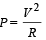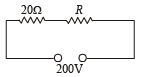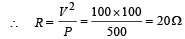NOTE : For the bulb to deliver 500 W, it should have a p.d. of 100 V across it. This would be possible only when R = 20 Ω  is in series with the bulb because in that case both resistances will share equal p.d.

Q.2. The equivalent resistance between points A and B of the circuit given below is ......Ω .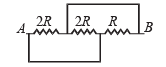Ans. R/2

Solution.  The given circuit may be redrawn as shown in the figure.

Thus, the resistances 2R, 2R and R are in parallel.

Hence,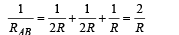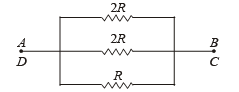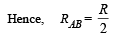Q.3. In the circuit shown below, each battery is 5V and has an internal resistance of 0.2 ohm.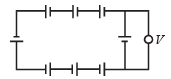The reading in the ideal voltmeter V is ..... V.

Ans. 0

Solution. Let a current I flow through the circuit. Net emf of the circuit = 8 (5V) = 40 V

Net resistance in the circuit = 8 (0.2 Ω) = 1.6 Ω Current flowing through the circuit,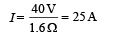The voltmeter reading would be V = E – IR  = (5V) – (25A) (0.2  Ω)

= 5V – 5V = 0

True/ False

Q.1. In an electrolytic solution the electric current is mainly due to the movement of free electrons.

Ans. F

Solution. NOTE : An electrolyte solution is formed by mixing an electrolyte in a solvent. The electrolyte on dissolution furnishes ions. The preferred movement of ions under the influence of electric field is responsible for electric current.

Q.2. Electrons in a conductor have no motion in the absence of a potential difference across it.

Ans. f

Solution. NOTE : Billions of electrons in a conductor are free and have thermal velocities. The electrons have motion in random directions even in the absence of potential difference.

Q.3. The current –voltage graph s for a given metallic wire at two different temperatures T1 and T2 are shown in the figure.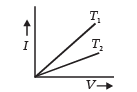The temperature T2 is greater than T1.

Ans. T

Solution.  For a given voltage, current is more in case of T1.

Since, V = IR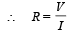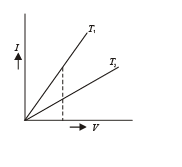Resistance is less in case of T1 and more in T2.

NOTE : For a metallic wire, resistance increases with temperature, therefore T2 > T1

Offer running on EduRev: Apply code STAYHOME200 to get INR 200 off on our premium plan EduRev Infinity!

42 docs|19 tests

,

,

,

,

,

,

,

,

,

,

,

,

,

,

,

,

,

,

,

,

,

,

,

,

,

,

,

,

,

,

,

,

,

;